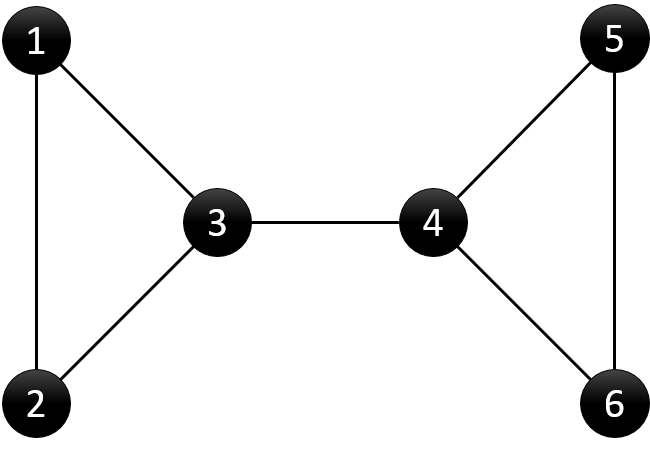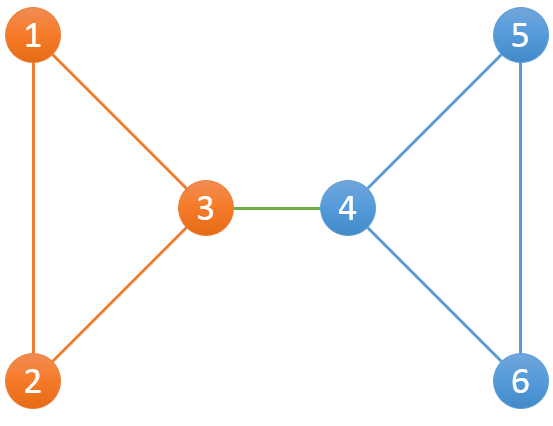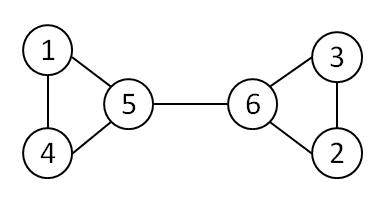# hihoCoder 1184-连通性二·边的双连通分量

hihoCoder 1184-连通性二·边的双连通分量
#1184 : 连通性二·边的双连通分量6 7
1 2
1 3
2 3
3 4
4 5
4 6
5 6

2
1 1 1 4 4 4

```#include<iostream>
#include<cstdio>
#include<stack>
#include<algorithm>
#include<vector>
using namespace std;
const int kMaxN = 20005;
int n, m;
vector<vector<int>> graph;
stack<int> stk;
int visit[kMaxN];
int dfn[kMaxN];
int low[kMaxN];
int parent[kMaxN];
int belong[kMaxN];
int bridge_num=0;
void dfs(int u) {
//记录dfs遍历次序
static int counter = 0;
//记录节点u的子树数
int children = 0;
visit[u] = 1;
//初始化dfn与low
dfn[u] = low[u] = ++counter;
//将u加入栈
stk.push(u);
for (int i = 0; i < graph[u].size(); i++) {
int v = graph[u][i];
//节点v未被访问，则(u,v)为树边
if (visit[v] == 0) {
children++;
parent[v] = u;
dfs(v);
low[u] = min(low[u], low[v]);
//bridge
if (low[v] > dfn[u]) {
bridge_num++;
}
}
//节点v已访问，则(u,v)为回边
else if (v != parent[u]) {
low[u] = min(low[u], dfn[v]);
}
}
if (low[u] == dfn[u])
{
int min_id = u;
vector<int> member;
while (stk.top() != u)
{
min_id = min(min_id, stk.top());
member.push_back(stk.top());
stk.pop();
}
member.push_back(u);
stk.pop();
for (int i = 0; i < member.size(); i++)
belong[member[i]] = min_id;
}
}
int main()
{
//freopen("input.txt", "r",stdin);
scanf("%d %d", &n, &m);
graph.resize(n + 1);
int u, v;
while (m--)
{
scanf("%d %d", &u, &v);
graph[u].push_back(v);
graph[v].push_back(u);
}
dfs(1);
printf("%dn", bridge_num+1);
for (int i = 1; i <= n; i++)
printf("%d ", belong[i]);
return 0;
}
```This site uses Akismet to reduce spam. Learn how your comment data is processed.